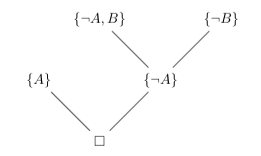# Resolution

Resolution is a single rule refutation technique for deciding the satisfiability of propositional formulas in clausal form.

Consider two clausal forms `A` and `B`. If there is a literal, `L`, such that `L``A` and `¬L``B`, then the clause (`A`-{`L`}) ∪ (`B`-{`¬L`}) is called the resolvent of `A` and `B` with respect to `L`. Given a set of clauses, resolution is the repeated generation of resolvents using the set elements or previously generated resolvents. This process continues until either the empty clause is generated or no more new resolvents can be created.

It can be shown that the resolvent of two clausal forms is a logical consequence of those forms. From this we can show that any number of applications of the resolution rule is sound by induction on the number of applications. We can also show that resolution is complete. So, if a clausal form is unsatisfiable, resolution will derive the empty clause (the clause which cannot be satisfied). As resolution is a refutation technique, our goal will be to derive the empty clause.

We can see a propositional derivation of a clause `C` from a set of clauses `S` using resolution as the forming of a tree `T` such that

1. `C` is the root of `T`,
2. every leaf of `T` belongs to `S`,
3. every non-leaf node `N` has children `N1` and `N2` and `N` is the resolvent of `N1` and `N2`.

The following is such a tree which shows that the set of clauses `{{A}, {¬A, B}, {¬B}}` derives the empty clause, `◻`, which means the set is unsatisfiable.## Algorithm

The resolution algorithm involves the repeated generation of resolvents until the empty clause is generated which would indicate success. Failure is indicated when all possible resolvents have been formed without generating the empty clause.

## Implementation

The following Prolog code can be found in the examples directory of the Barry's Prolog distribution.

First we fix a set of operators which we will need to represent the formulas of propositional logic.

``` :-op(200, fy, ~). % Negation :-op(0, yfx, (/\)). :-op(400, xfy, (/\)). % Conjunction (not ISO associativity which is yfx) :-op(0, yfx, (\/)). :-op(500, xfy, (\/)). % Disjunction (not ISO associativity which is yfx) :-op(600, xfy, =>). % Implication :-op(700, xfy, <=>). % Equivalence ```

The procedure `pl_negate(A, B)` succeeds if A is the negation of B.

``` pl_negate(true, false) :- !. pl_negate(false, true) :- !. pl_negate(~A, A) :- !. pl_negate(A, ~A). ```

The procedure `pl_resolve(ClausesIn, PairsIn, PairsOut, ClausesOut)` succeeds if a propositional resolvent can be formed from two clauses in `ClausesIn`. `ClausesOut` is `ClausesIn` with the resolvent added. We take care here to not form resolvents we have already formed as this would be a waste of effort. The `PairsIn` argument is a list of sorted pairs of clauses that have been used to generate a resolvent. The `PairsOut` argument is `PairsIn` with the new pair added to the head of the list.

``` pl_resolve(ClausesIn, PairsIn, PairsOut, ClausesOut) :- member(A, ClausesIn), member(Atom, A), pl_negate(Atom, NegAtom), member(B, ClausesIn), member(NegAtom, B), (A @< B, \+ member(A-B, PairsIn), PairsOut = [A-B|PairsIn] ; A @>= B, \+ member(B-A, PairsIn), PairsOut = [B-A|PairsIn]), ord_subtract(A, [Atom], RestA), ord_subtract(B, [NegAtom], RestB), ord_union(RestA, RestB, Resolvent), ord_union([Resolvent], ClausesIn, ClausesOut), !. ```

The procedure `pl_resolution_loop(Clauses)` succeeds if `Clauses` can derive the empty clause which would be a refutation. The empty clause is just the empty list - `[]`. Basically, we just keep applying the resolution rule until we cannot apply it any more.

``` pl_resolution_loop(Clauses, _) :- member([], Clauses), !. pl_resolution_loop(Clauses, Pairs) :- pl_resolve(Clauses, Pairs, NewPairs, NewClauses), pl_resolution_loop(NewClauses, NewPairs). ```

The procedure `pl_remove_all_false_atoms(ClausesIn, ClausesOut)` removes all of the `false` atoms from the clauses. `ClausesOut` is the clausal form `ClausesIn` where all `false` atoms have been removed. The `false` atom has no meaning for us in a disjunction. Remember that `(A \/ false) <=> A`. Getting rid of them before we search for a refutation would save time and energy.

``` pl_remove_all_false_atoms([], []) :- !. pl_remove_all_false_atoms([H|T], [NewH|NewT]) :- ord_subtract(H, [false], NewH), pl_remove_all_false_atoms(T, NewT). ```

The procedure `pl_resolution(Formula)` Succeeds if `Formula` can be refuted. We first translate into conjunctive normal form (CNF), then into clausal form, then we remove all `false` atoms. We are then ready to resolve with `pl_resolution_loop/2`

``` pl_resolution(Formula) :- cnf_transform(Formula, CNF), pl_clausal_form(CNF, Clauses0), pl_remove_all_false_atoms(Clauses0, Clauses1), pl_resolution_loop(Clauses1, []). ```

The procedure `pl_resolution_tautology(Formula)` succeeds if `Formula` is a tautology. As resolution is a refutation technique, we succeed if the negation of `Formula` derives the empty clause.

``` pl_resolution_tautology(Formula) :- pl_resolution(~Formula). ```

## References

Doets, Kees From Logic to Logic Programming. MIT Press, 1994.